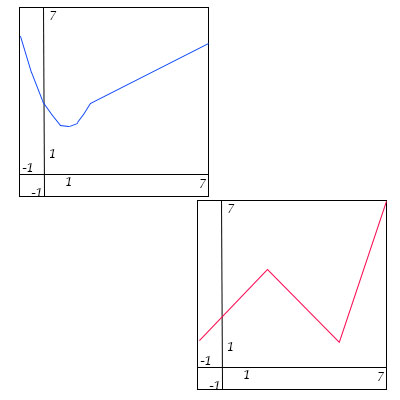# The graphs of the function F (left, in blue) and G (right, in red) are below. Let P(x) = F(x)G(x)...

## Question:

The graphs of the function {eq}F {/eq} (left, in blue) and {eq}G {/eq} (right, in red) are below. Let {eq}P(x) = F(x)G(x) {/eq} and {eq}Q(x) = \frac{F (x)}{G(x)} {/eq}. Determine: {eq}P'(1), \ Q'(1), \ P'(6), \ Q'(6) {/eq}## Product and Quotient Rule of Differentiation:

If the two functions {eq}f(x) {/eq} and {eq}g(x) {/eq} are differentiable (i.e. the derivative exist) then the product is differentiable and,

{eq}{\left( {f\,g} \right)^\prime } = f'\,g + f\,g' {/eq}

If the two functions {eq}f(x) {/eq} and {eq}g(x) {/eq} are differentiable (i.e. the derivative exist) then the quotient is differentiable and,

{eq}{\left( {\frac{f}{g}} \right)^\prime } = \frac{{f'\,g - f\,g'}}{{{g^2}}} {/eq}

From the graph, it is observed that {eq}F(1)=2 {/eq} and {eq}G(1)=2 {/eq}.

The slope of the function {eq}F(x) {/eq} at {eq}x=1 {/eq} is {eq}0 {/eq}.

Therefore, {eq}F'(1)=0 {/eq}.

The point {eq}(0,1) {/eq} and {eq}(1,2) {/eq} lies on the graph of {eq}G(x) {/eq}.

The slope of the function {eq}G(x) {/eq} at {eq}x=1 {/eq} is {eq}\frac{2-1}{1-0}=1 {/eq}.

Therefore, {eq}G'(1)=1 {/eq}.

From the graph, it is observed that {eq}F(6)=5 {/eq} and {eq}G(6)=2 {/eq}.

The point {eq}(4,4) {/eq} and {eq}(6,5) {/eq} lies on the graph of {eq}F(x) {/eq}.

The slope of the function {eq}F(x) {/eq} at {eq}x=6 {/eq} is {eq}\frac{5-4}{6-4}=\frac{1}{2} {/eq}.

Therefore, {eq}F'(6)=\frac{1}{2} {/eq}.

The point {eq}(5,0) {/eq} and {eq}(6,2) {/eq} lies on the graph of {eq}G(x) {/eq}.

The slope of the function {eq}G(x) {/eq} at {eq}x=6 {/eq} is {eq}\frac{2-0}{6-5}=2 {/eq}.

Therefore, {eq}G'(6)=2 {/eq}.

a)

{eq}P'(1)=(F'(1))(G(1))+(F(1))(G'(1))=(0)(2)+(2)(1)=2 {/eq}

b)

{eq}Q'(1)=\left(\frac{(G(1))(F'(1))-(F(1))(G'(1))}{(G(1))^2} \right)=\frac{(2)(0)-(2)(1)}{2^2}=-\frac{2}{4}=-\frac{1}{2} {/eq}

c)

{eq}P'(6)=(F'(6))(G(6))+(F(6))(G'(6))=\left(\frac{1}{2}\right)(2)+(5)(2)=11 {/eq}

d)

{eq}Q'(6)=\left(\frac{(G(6))(F'(6))-(F(6))(G'(6))}{(G(6))^2} \right)=\frac{(2)\left(\frac{1}{2}\right)-(5)(2)}{2^2}=-\frac{9}{4} {/eq}